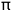<-- Back to Previous Page TOC Next Section -->Section 4.1: Introduction to Sound Synthesis

What Is Sound Synthesis?

We’ve learned that a digitally recorded sound is stored as a sequence of numbers. What would happen if, instead of using recorded sounds to generate those numbers, we simply generated the numbers ourselves, using the computer? For instance, what if we just randomly select 44,100 numbers (1 second’s worth) and call them samples? What might the result sound like?

The answer is noise. No surprise here—it makes sense that a signal generated randomly would sound like noise. Philosophically (and mathematically), that’s more or less the definition of noise: random or, more precisely, unpredictable information. While noise can be sonically interesting (at least at first!) and useful for lots of things, it’s clear that we’ll want to be able to generate other types of sounds too. We’ve seen how fundamentally important sine waves are; how about generating one of those? The formula for generating a sine wave is very straightforward:

y = sin(x)

That’s simple, but controlling frequency, amplitude, phase, or anything more complicated than a sine wave can get a bit trickier.Applet 4.1
Bring in da funk,
bring in da noise

This applet allows you to hear some basic types of noise: pink, white, and red.

We use different colors to describe different types of noise, based on the average frequency content of the noiseband. White noise contains all frequencies, at random amplitudes (some of which may be zero). Pink noise has a heavier dose of lower frequencies, and red noise even more.

Sine Wave Example

If we take a sequence of numbers (the usual range is 0 to 2, perhaps with a phase increment of 0.1—meaning that we’re adding 0.1 for each value) and plug them into the sine formula, what results is another sequence of numbers that describe a sine wave. By carefully selecting the numbers, we can control the frequency of the generated sine wave.

Most basic computer synthesis methods follow this same general scheme: a formula or function is defined that accepts a sequence of values as input. Since waveforms are defined in terms of amplitudes in time, the input sequence of numbers to a synthesis function is usually an ongoing list of time values. A synthesis function usually outputs a sequence of sample values describing a waveform. These sample values are, then, a function of time—the result of the specific synthesis method.

For example, assume the following sequence of time values.

(t1, t2, t3,....., tn)

Then we have some function of those values (we can call it f(t)):

(f(t1), f(t2), f(t3),....., f(tn)) = (s1, s2, s3,....., sn)

(s1, s2, s3,....., sn) are the sample values.

This sine wave function is a very simple example. For some sequence of time values (t1, t2, t3,....., tn), sin(t) gives us a new set of numbers {sin(t1), sin(t2), sin(t3),....., sin(tn)}, which we call the signal’s sample values.Xtra bit 4.1
Computer code
for generating
an array of
a sine wave

As we’ll see later, more sophisticated functions can be used to generate increasingly complex waveforms. Often, instead of generating samples from a raw function in time, we take in the results of applying a previous function (a list of numbers, not a list of time values) and do something to that list with our new function. In fact, we have a more general name for sound functions that take one sequence of numbers (samples) as an input and give you another list back: they’re called filters.Xtra bit 4.2
Composer Robert MarsanyiXtra bit 4.3
Early computer music network ensembles

You may never have to get quite this "down and dirty" when dealing with sound synthesis—most computer music software takes care of things like generating noise or sine waves for you. But it’s important to understand that there’s no reason why the samples we use need to come from the real world. By using the many available synthesis techniques, we can produce a virtually unlimited variety of purely synthetic sounds, ranging from clearly artificial, machine-made sounds to ones that sound almost exactly like their "real" counterparts!

There are many different approaches to sound synthesis, each with its own strengths, weaknesses, and suitability for creating certain types of sound. In the sections that follow, we’ll take a look at some simple and powerful synthesis techniques.

 <-- Back to Previous Page Next Section -->